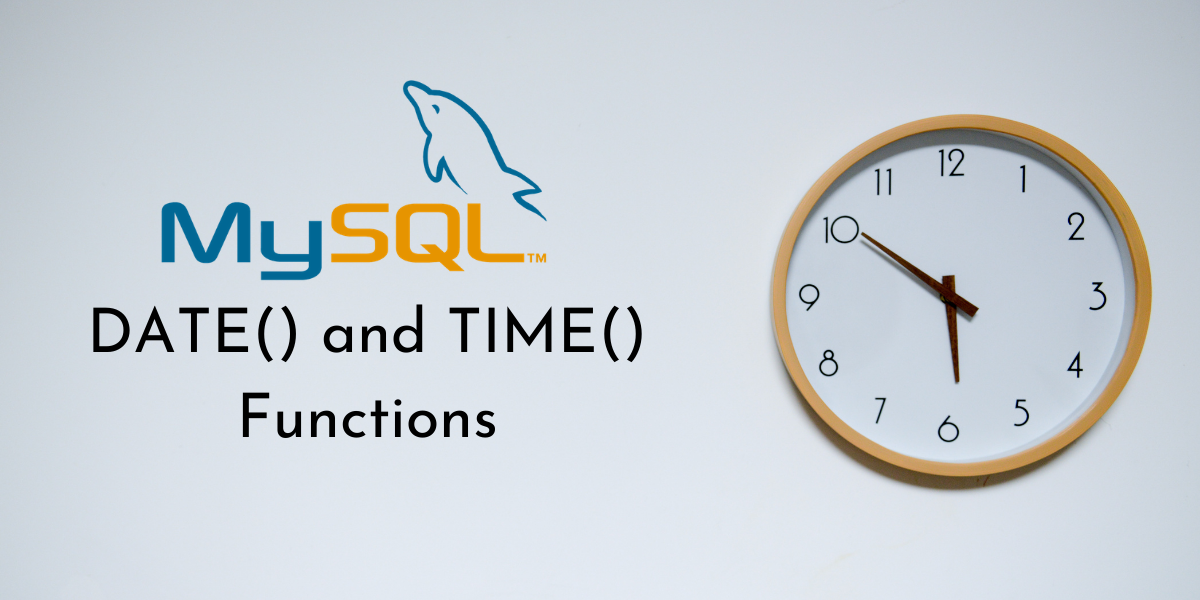# MySQL DATE() and TIME() – How to display the date and time in MySQL?In this tutorial, we will study the MySQL `DATE()` and `TIME()` functions. So far, we have seen functions like `CURDATE()`, `CURTIME()` and `NOW()` that help display and store the current date, time, and DateTime values respectively. But what if you already have those values stored in a table and want to display them?

Suppose you have a column that stores the date and the time in the format YYYY-MM-DD HH:MM:SS. One way to display this column would be to use the `SELECT` statement. However, we want to return a more detailed output such that the date part of the value and column part of the value in the column is printed as two separate columns in the result-set. This means we are extracting the date and the time separately from a DateTime value. For this operation, MySQL provides us with the `DATE()` and `TIME()` functions.

• The `DATE()` function is used to extract the date from a date or DateTime value.
• The `TIME()` function is used to extract the time from a time or DateTime value.

## Syntax of MySQL DATE()

```DATE(expression)
```

Where ‘expression’ is a valid date or datetime value. If we pass any other kind of value, the function returns NULL.

## Syntax of MySQL TIME()

```TIME(expression)
```

Where ‘expression’ is a valid time or datetime value. If we pass any other kind of value, the function returns NULL.

## Examples of MySQL DATE() and TIME()

Let us kick things off with some basic examples. Suppose you have the following datetime value – 2020-06-16 12:45:05. Let us use the `DATE()` function to extract the date from the given value and display it. We will use the `SELECT` statement and aliases for this. The query is –

```SELECT DATE('2020-06-16 12:45:05') AS Date;
```

And the output is –

Now, let us extract the time value from the given datetime value using the `TIME()` function. The query is –

```SELECT TIME('2020-06-16 12:45:05') AS Time;
```

And the output is –

### Using DATE() and TIME() with the NOW() function

Let us explore the DATE() and TIME() more using the NOW() function. Yes, we can pass functions like NOW() to DATE() and TIME() functions since NOW() returns a datetime value. Consider the below query.

```SELECT NOW(), DATE(NOW()), TIME(NOW());
```

And the output is –

### Passing a date value to DATE()

Apart from a datetime value, we can also pass a date value to the MySQL DATE() function. The query is –

```SELECT DATE('2021-05-06') AS Date;
```

And the output is –

### Passing a time value to TIME()

Apart from a datetime value, we can also pass a time value to the MySQL TIME() function. Let us make things interesting by passing a time value with fractional seconds precision. The query is –

```SELECT TIME('05:41:06.000543') AS Time;
```

And the output is –

### DATE() and TIME() Example With Tables

Consider the below Employee table.

Let us write a query that displays the employee id, employee name, their check-in date as the CheckInDate column and the check-in time as the CheckInTime column in the result-set. We will extract the date and time values from the CheckInDateTime column of the Employee table. The query is –

```SELECT eid, Name,
DATE(CheckInDateTime) AS CheckInDate,
TIME(CheckInDateTime) AS CheckInTime
FROM Employee;
```

And the output is –

## Conclusion

You will find yourself using the MySQL `DATE()` and `TIME()` functions quite often while working with databases. Extracting the date and time from tables and databases is a fairly common operation used in many day-to-day databases. I would highly encourage you to practice these functions.